# What is QuickSort in Data Structure?

Hello, In this article you are going to understand the basic concept of QuickSort Algorithm, how it works internally and then what is the algorithm of Quicksort. (Youtube Link – Quick Sort)

## Points of Interest

• This algorithm is also based on Divide and Conquer approach. Sometimes it is also called as partition-exchange sort.
• It is 2-3 times faster than its competitors Merge and Heap Sort when implemented well
• An element from the list is selected known as ‘pivot’ and partitioning of the list is based on that pivot. This process — selecting of pivot and then partitioning is continue to occur until there is one element left which cannot be divided further.
• There are several methods for the selection of pivot and steps required for partitioning. They should be choose wisely as it has a great effect on its algorithm’s performance.

## Performance of QuickSort

The Worst Case of Quicksort is : O(n^2)
It’s Best and Average Case is same i.e., : O(nlogn)

## Pivot Selection

As I told you there are several ways to select pivot which has a great impact on the performance of its algorithm. You can select the first element of list as pivot, or the last one, or it can be any random element in the list. So if you go for random selection then it is called ‘Randomized Quicksort’

## How Quicksort Works

In this sorting, first the array is divided into 2 sub-arrays based on the pivot value. one of smaller elements than pivot and other containing elements greater than pivot. Then recursively it sort the sub-arrays.

## Step by Step Example

Consider taking an array containing unordered integer values. Suppose we have to sort it in ascending order, So let’s do it with the help of Quicksort.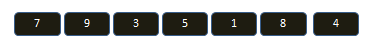Step 1 : Pick last element as PIVOT, and put all the smallest elements of the list to its LEFT and the greatest elements towards its RIGHT. (PIVOT element is shown in RED box)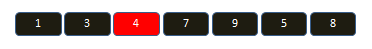NOTE : Ordering of all left and right elements of the pivot, does not matters.

Step 2 : Divide the list to two sub-lists, one containing all the smalls and other containing all the greater. Repeat the whole process recursively till only 1 or no element left.(Exit condition of loop)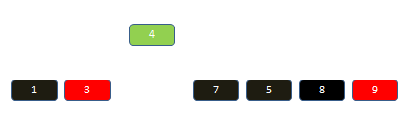Step 3 :Previous PIVOT elements are shown in Green box, Note that they are placed on their correct index position in this process.Step 4 : Pivot ->3 will have only 1 sublist as it no greater elements than pivot.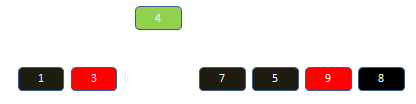Step 5 :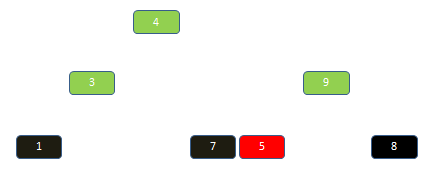Step 6 :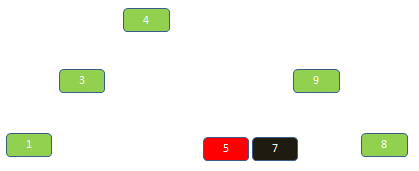Step 7 :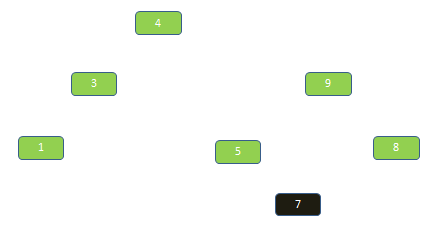Step 8 :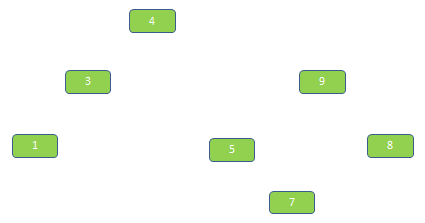Step 9 : Sorted List obtained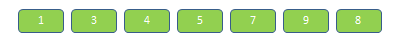Watch this video of Quick Sort